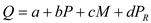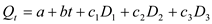## Quiz 7 : Demand Estimation and ForecastingLooking for Economics Homework Help?# Quiz 7 : Demand Estimation and Forecasting

The following is the demand equation of W Company that sells tennis ball:Where, P = price of tennis ball, Q = quantity demanded, M = average income of consumers and P R = price of related goods. a. The quantity demanded ( Q ) depended upon following factors: • Intercept " a " denotes the quantity demanded when all other factors are zero. • " b " is negative and states that price is negatively related to quantity. • " c " is positively related to quantity as increase in income increases the quantity demanded. • " d " is slope of related goods that explains as price of related goods increase, the quantity demanded falls. • All the intercept and coefficients have a significant p-value. This is because the p-value. Is almost equal to 0 b. The estimated number of tennis balls can be obtained by substituting the values of variables:The estimated quantity demanded is 240,134. c. The value of price elasticity of demand ( E ) is,The value of income elasticity of demand ( E M ) is,The value of price of related goods elasticity of demand or cross price elasticity of demand ( E R ) is,d. Given the price elasticity of demand is 0.256, the percentage change in quantity for 15% change in price would be:If the price decreases by 15%, the quantity demanded would increase by 3.84%. e. Given the income elasticity of demand is 00.15, the percentage change in quantity for 20% increase in income would be:If income increases by 20%, the quantity increases by 3%. f. Given the cross elasticity of demand is -0.667, the percentage change in quantity for 25% increase in price of racket would be:If the price of racket increases by 25%, then its quantity demanded would decreases by 0.1667%.

CR's sales vary seasonally. a. If seasonal variation is not considered while estimating the demanded quantity, then the results derived from estimation would be misleading. • There would be large standard errors and variance. • The unexplained error ratio R 2 would be high. • The p-value of variables might be different than zero and significant. b. Dummy variable is included when there are seasonal or cyclical fluctuations. The value of dummy variable can be 0 or 1. • If dummy variable is included when it is not required, then the standard error may be slightly high than usual. • However, this won't give misleading results as t-test will make the variables statistically insignificant.

The following equation shows the sales forecast of athletic shoes:Where, Q t = sales of t quarter and all Ds are dummy variables of seasonal fluctuation in sales. a. As the intercept and coefficients of all dummy variables have a positive value, it could be said that there is an upward sales trend. All variables are positively related to sales. b. Looking upon the p-value, one can say that the calculated t value would be certainly higher than the given p-value. This means the seasonal patterns are statically significant. c. To arrive at the estimated sales of quarter of 2014(III) and 2015(II), substitute the value of intercept and coefficient in the demand equation. • The value of t would be 31 for 2014(III):• The value of t would be 34 for 2015(II):• Among spring and summer, the sale is highest in summer. d. The forecast equation can be improved by adding other important variables such as: • Price of the related goods such as socks. • Income of the consumers. • Number of competitors. • Price of substitute shoes.